# 978-0123869449 Chapter 12 Part 2

Document Type
Homework Help
Book Title
Authors
Michael F. Modest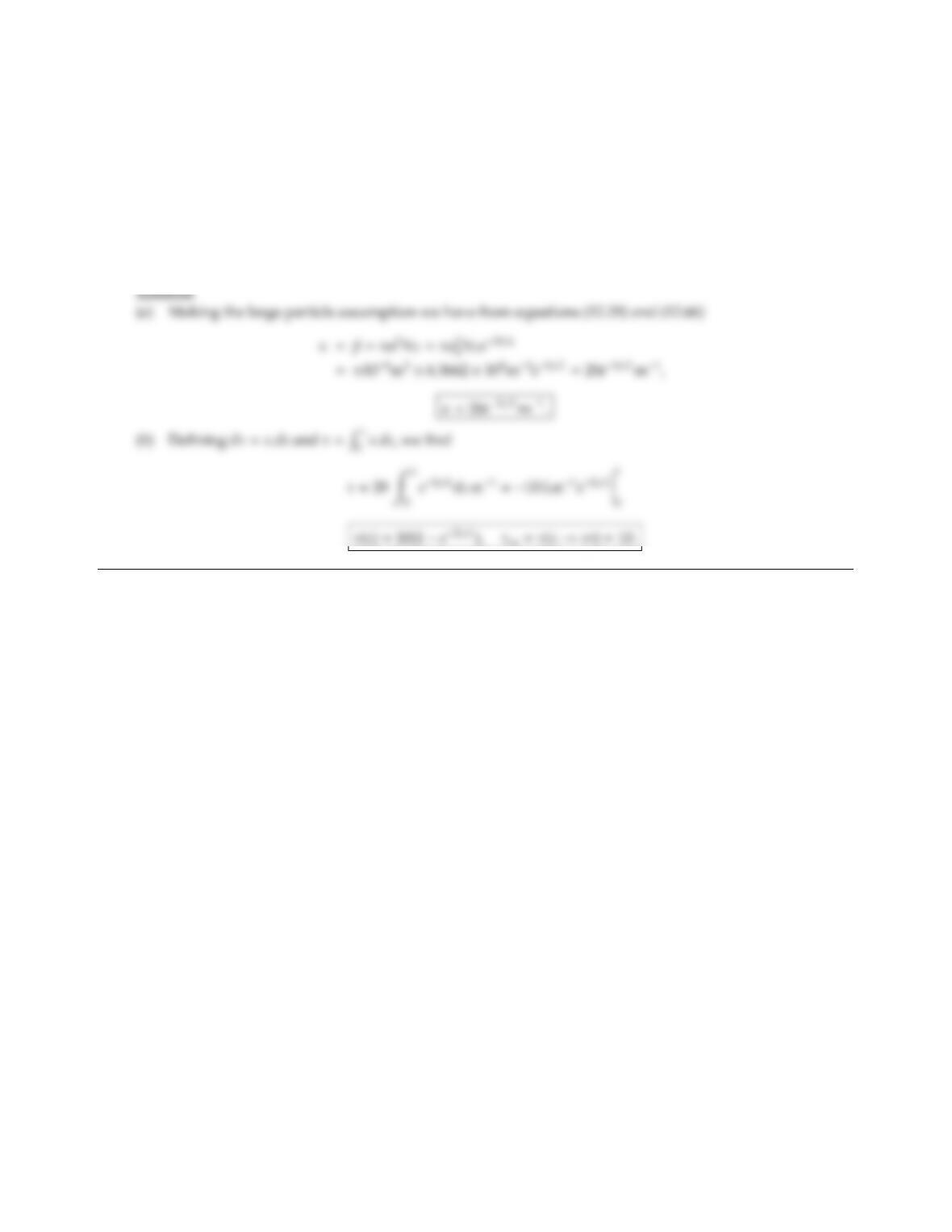12.13 A semi-infinite space is filled with black spheres. At any given distance, z, away from the plate the particle
number density is identical, namely NT=6.3662 ×108m3. However, the radius of the suspended spheres
diminishes monotonically away from the surface as
a=a0ez/L;a0=104m,L=1 m.
(a) Determine the absorption coecient as a function of z(you may make the large-particle assumption).
(b) Determine the optical coordinate as a function of z. What is the total optical thickness of the semi-infinite
space?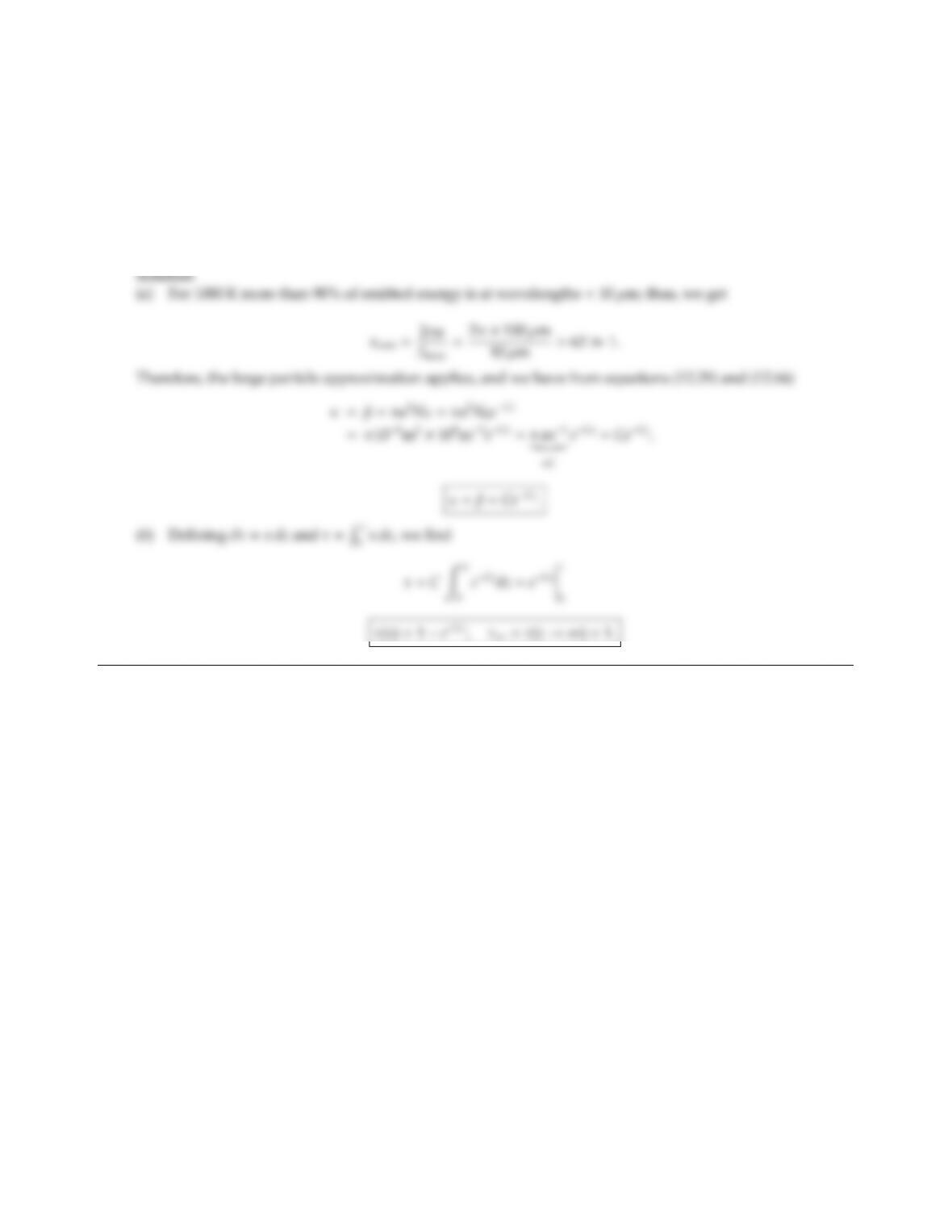CHAPTER 12 351
12.14 A semi-infinite space is filled with black spheres of uniform radius a=100 µm. The particle number density
is maximum adjacent to the surface, and decays exponentially away from the surface according to
NT=N0eCz;N0=108m3,C=πm1.
(a) Determine the absorption and extinction coecients as functions of z.
(b) Determine the optical coordinate as a function of z. What is the total optical thickness of the semi-infinite
space?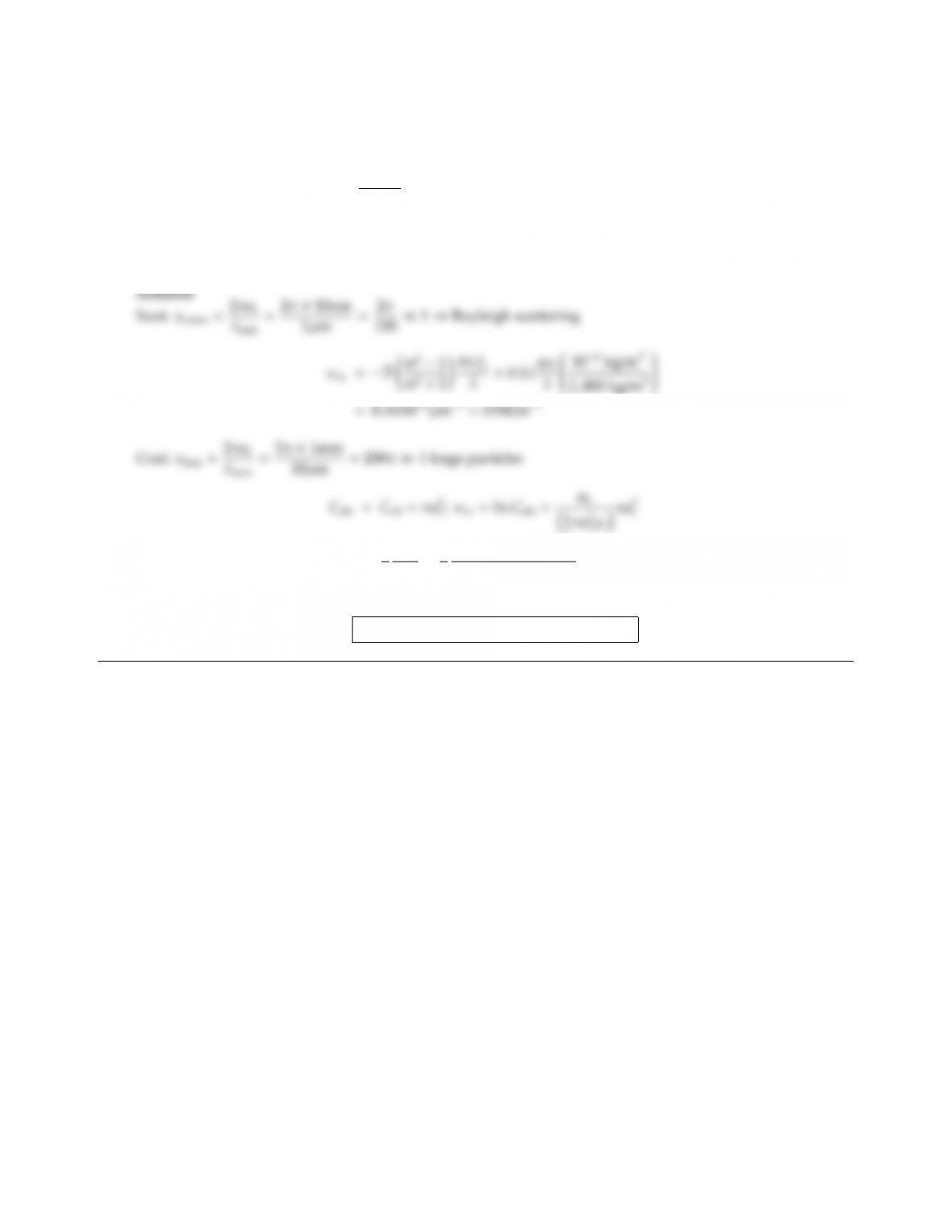12.15 In a combustion chamber radiatively nonparticipating gases are mixed with soot and coal particles. The
following is known (per m3of mixture):
Soot: uniform particle size, as=10 nm, mass =103kg,
complex index of refraction m21
m2+2=0.5λ20.1λi(λin µm).
Coal: uniform particle size, ac=1 mm, mass =1 kg, coal is black.
Density of both, coal and soot, is 2,000 kg/m3.
Determine the spectral absorption coecient of the mixture for the near infrared.
=3
4
mc
ρcac
=3
4
1 kg/m3
2000 kg/m3×1mm =0.375m1
Total absorption coecient:
κλ=κλs+κλc=0.942 +0.375 =1.317m1.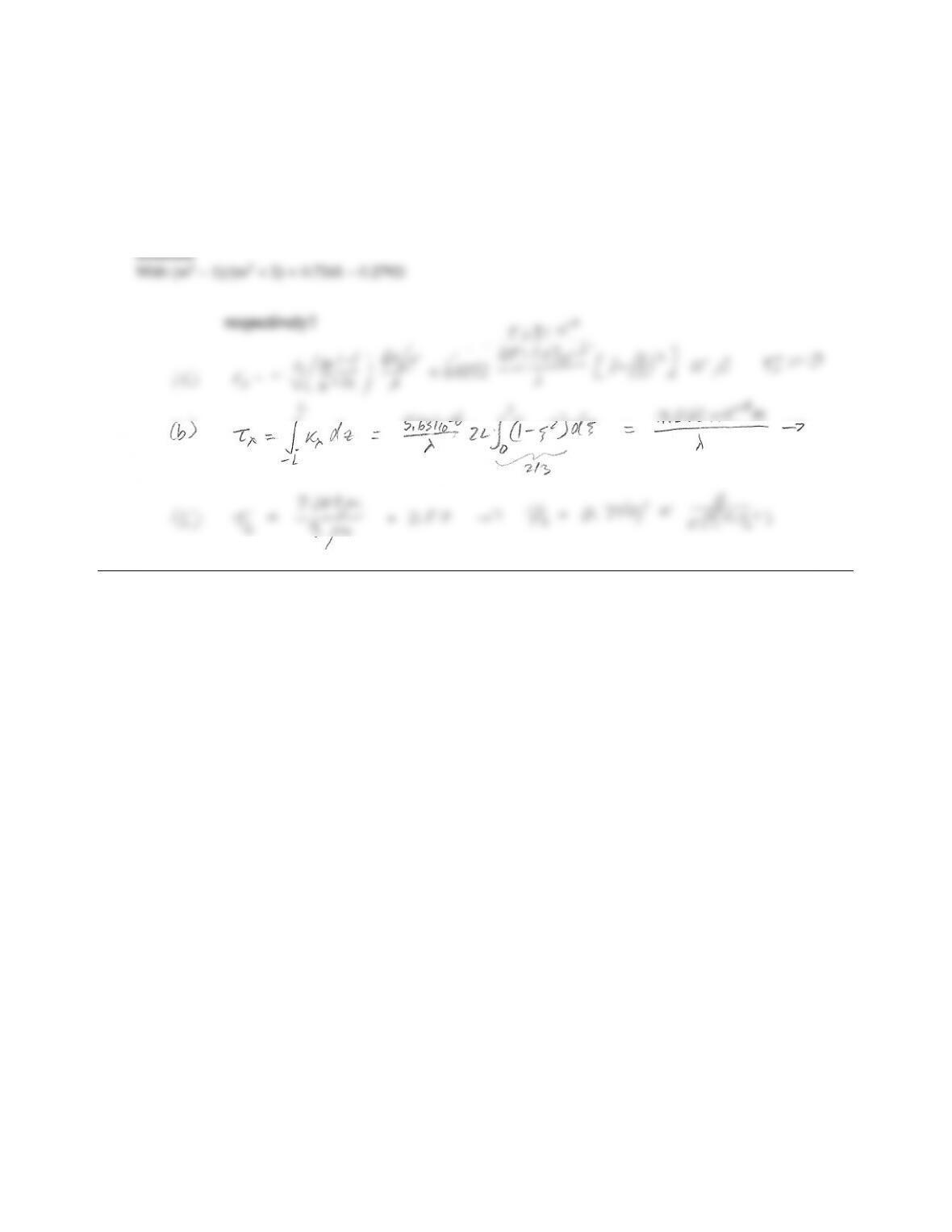CHAPTER 12 353
12.16 In a sheet ﬂame confined between two large parallel plates Lz+L=1 m soot is generated mainly in the
central ﬂame region, leading to a local soot volume fraction of fv(z)=fv0[1 (z/L)2], with fv0=1.07 ×106.
The soot is propane soot with a complex index of refraction of m=2.21 1.23i.
(a) Determine the relevant radiative properties of the mixture, assuming the combustion gases to be non-
participating.
(b) What is the spectral optical thickness of the 2Lthick layer?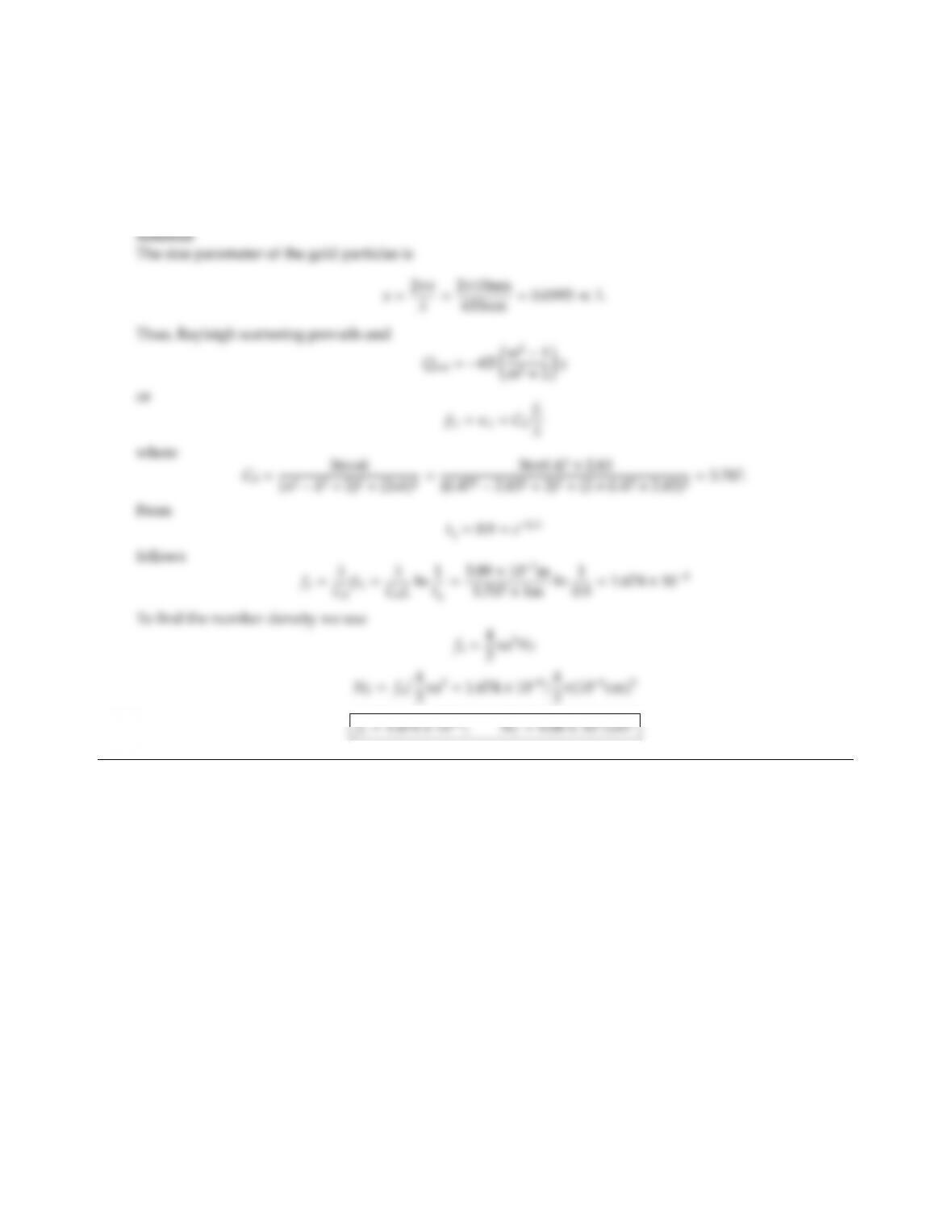12.17 A laser beam at 633 nm wavelength is probing a 1 m thick layer of gold nanoparticles suspended in air (radius
a=10 nm; for gold at 633 nm: m=0.47 2.83i). If the exiting laser beams is attenuated by 10% due to
absorption and scattering, determine
(a) the number density of gold particles,
(b) their volume fraction.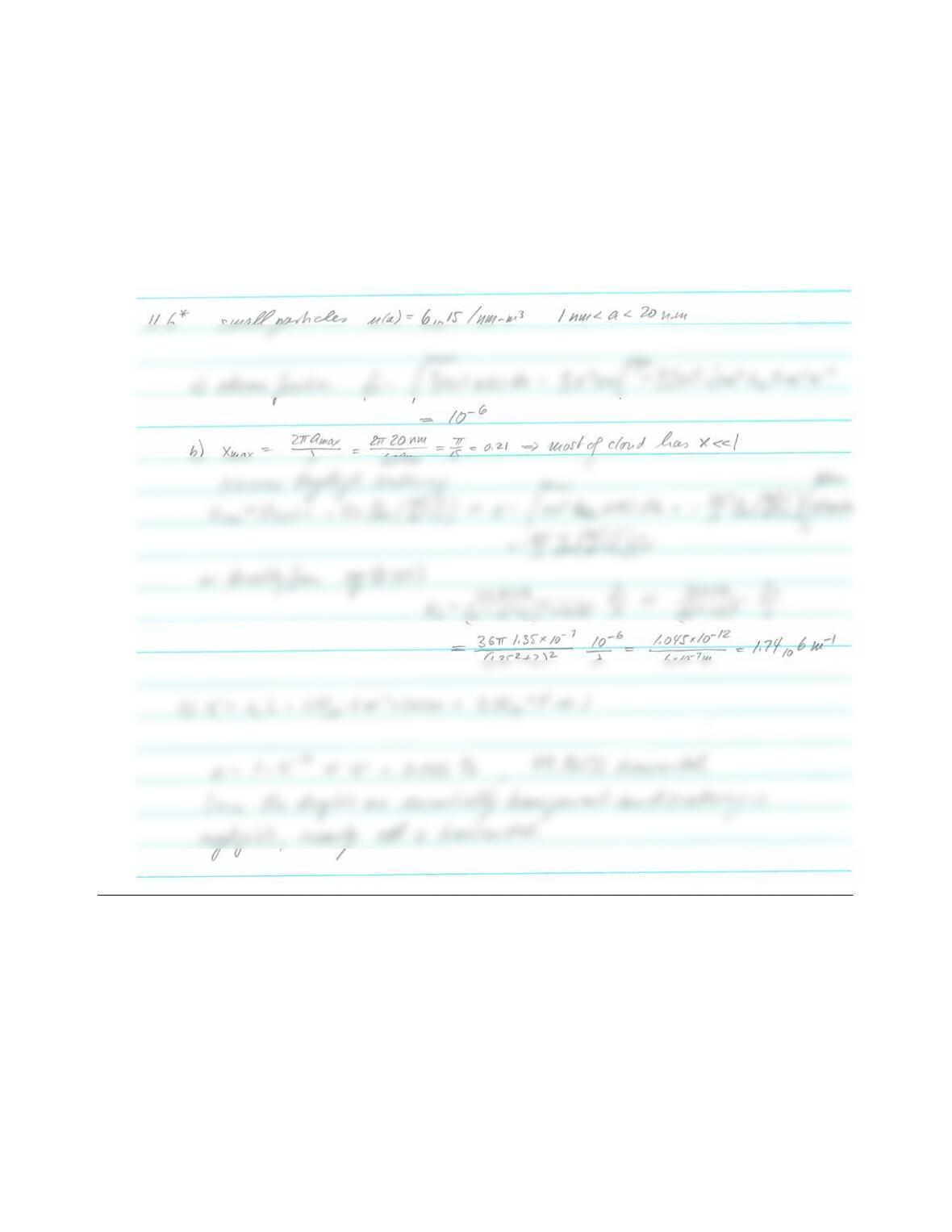CHAPTER 12 355
12.18 A LIDAR laser beam (operating in the green at λ=0.6µm) is shot into the sky. At a height of 1km the
laser encounters a 200m thick cloud consisting of tiny water droplets of varying size (1 nm a20 nm), but
constant particle distribution function everywhere (n=6×1015/nm m3).
1. Determine the water droplet volume fraction in the cloud.
2. Determine its spectral absorption coecient; compare with equation (12.123).
3. What fraction of the laser beam will be transmitted through the cloud? How much will be absorbed?
Note: Water at 0.6µm has an index of refraction of m1.35 107i.
Solution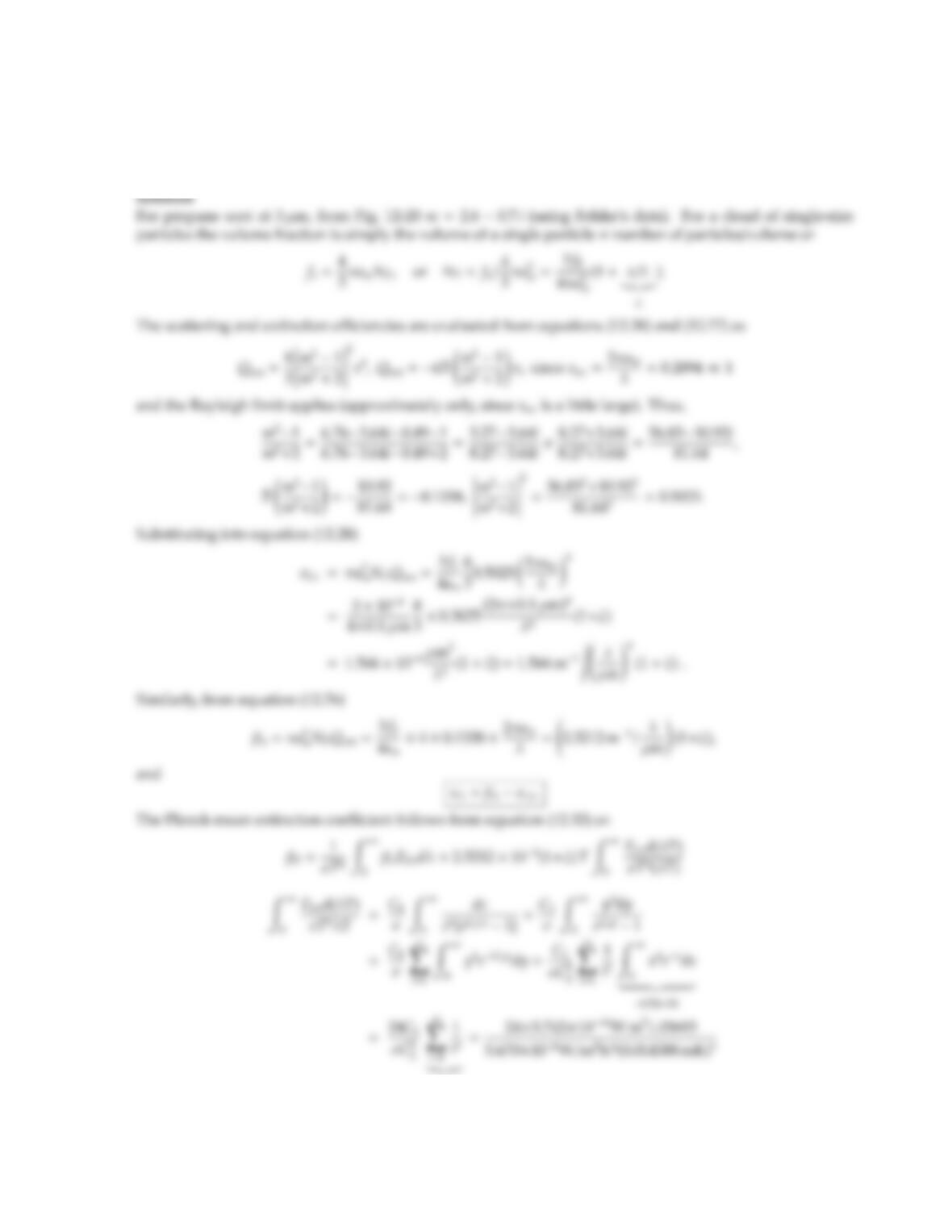12.19 Redo Problem 12.4 for propane soot with a single mean radius of am=0.1µm in a ﬂame with f0=106and
T0=1500 K. Show that the small particle limit is appropriate for, say, λ > 3µm. For hand calculations you
may approximate the index of refraction by a single average value (say, at 3 µm), and the emissive power by
Wien’s law.
=1.03693
=266.3 m1K1.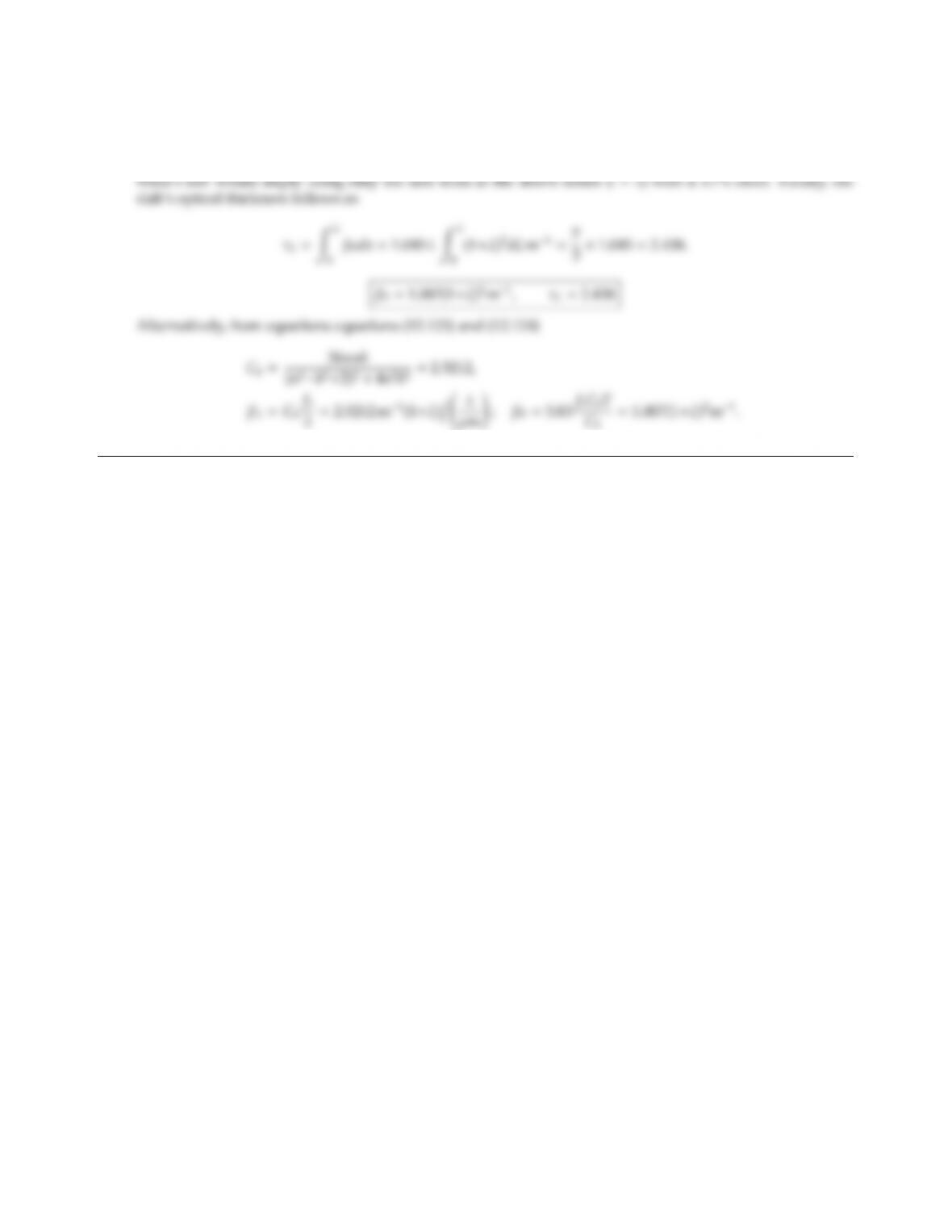CHAPTER 12 357
Thus,
βP=2.5212 ×106×266.3 m1K11500 K(1+ξ)2=1.007(1+ξ)2m1.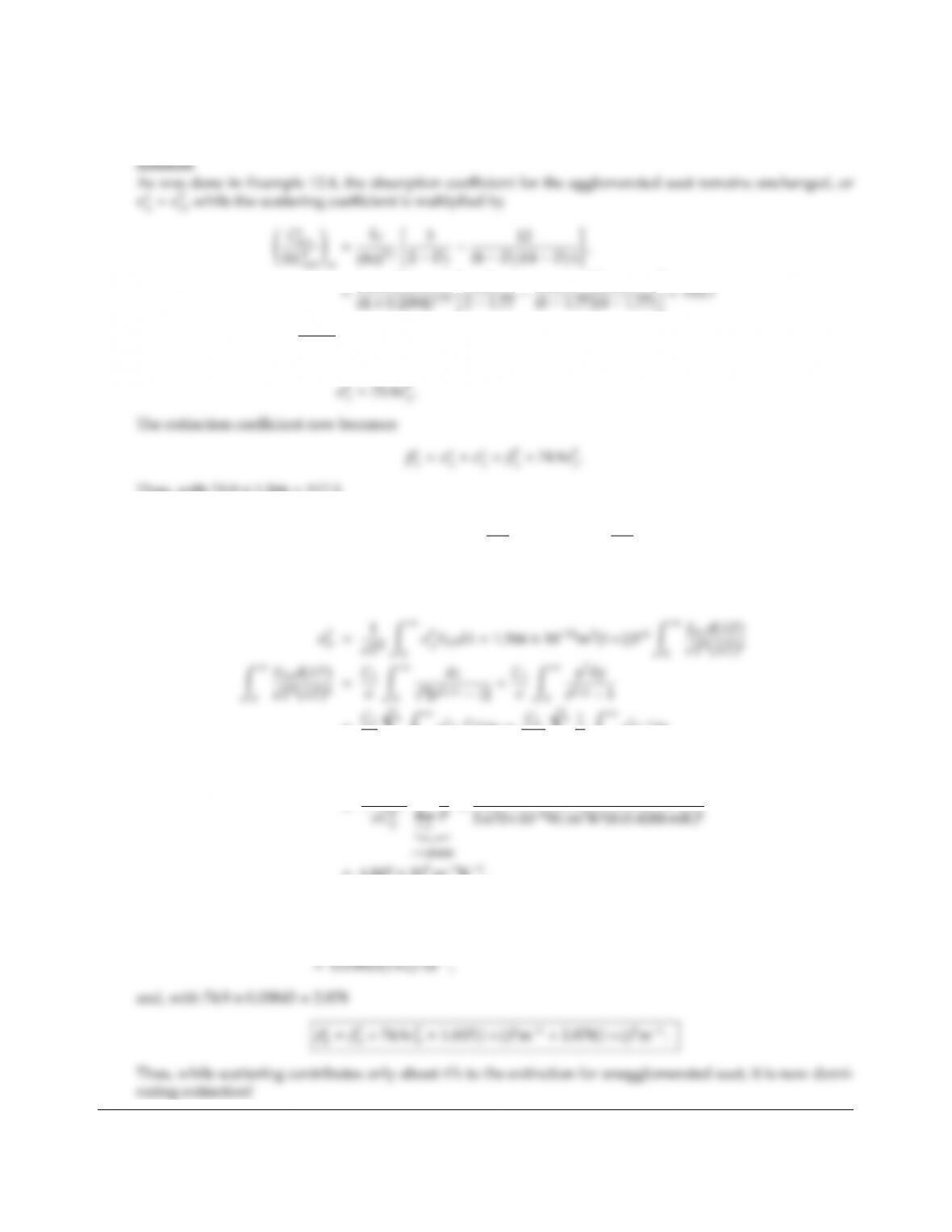12.20 Redo Problem 12.19 for the case that the soot has agglomerated into mass fractal aggregates of 1000 soot
particles each (Df=1.77 and kf=8.1).
Ca
sca
NCp
sca
=193.7110001/4=75.9
and
Thus, with 74.9×1.566 =117.3
βλ=2.521 m1(1+ξ), λ
µm!+117.3m1, λ
µm!4
.
To evaluate the Planck-mean extinction coecient, we first evaluate the Planck-mean scattering coecient
for single particles
=C1
σ
i=1Z
0
y7eiC2ydy =C1
σC5
2
i=1
i8Z
0
x7exdx
| {z }
(8)=1344
=1344C1
X
1
=1344×3.742×1016W m21.00408
=4.847 ×109m4K4.
Thus,
σp
P=1.566 ×1024m3(1+ξ)[1500 K(1+ξ)]44.847 ×109m4K4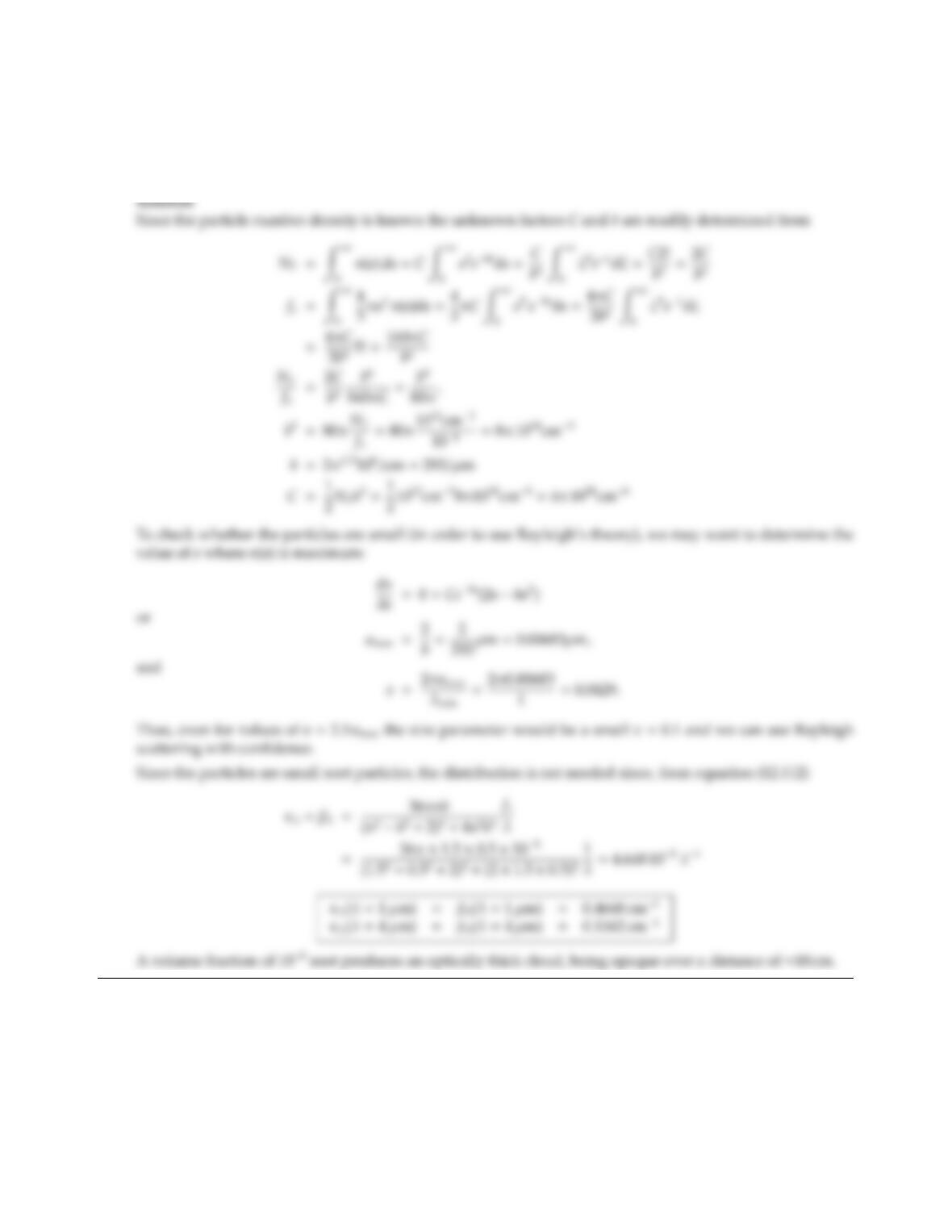CHAPTER 12 359
12.21 Consider a particle cloud with a distribution function of n(a)=Ca2eba, where ais particle radius and band
Care constants. The particles are soot (m1.50.5i), and measurements show the soot occupies a volume
fraction of 105, while the number density has been measured as NT=1012/cm3. Calculate the extinction,
absorption, and scattering coecients of the cloud for the wavelength range 1 µm<λ<4µm.

## Trusted by Thousands ofStudents

Here are what students say about us.